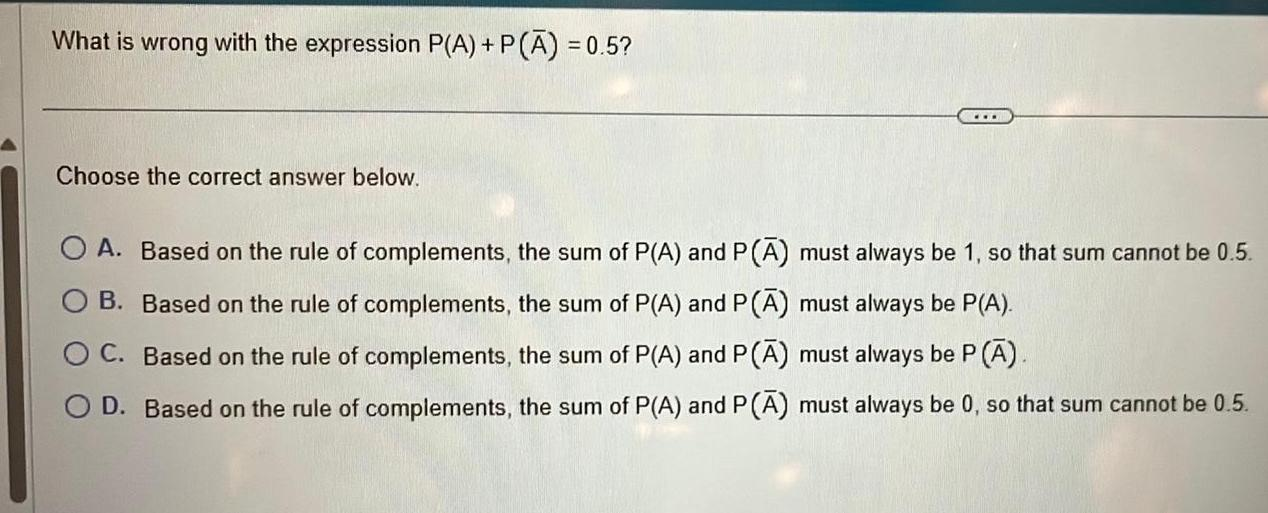Question:

# What is wrong with the expression P A P A 0 5 Choose the

Last updated: 11/20/2023What is wrong with the expression P A P A 0 5 Choose the correct answer below O A Based on the rule of complements the sum of P A and P must always be 1 so that sum cannot be 0 5 B Based on the rule of complements the sum of P A and P A must always be P A O C Based on the rule of complements the sum of P A and P A must always be P A O D Based on the rule of complements the sum of P A and P A must always be 0 so that sum cannot be 0 5